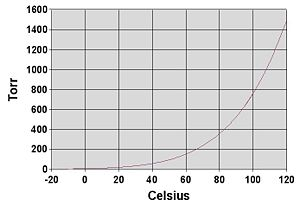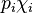# Vapor pressureGraph of water vapor pressure (in Torr) versus temperature (in Celsius). Note that at the boiling point of 100 °C, the vapor pressure equals the standard atmospheric pressure of 760 Torr.

Vapor pressure (or vapour pressure) is the pressure of a vapor in equilibrium with its non-vapor phases. Under appropriate conditions of temperature and pressure, solids and liquids tend to evaporate to a gaseous form, and gases tend to condense back. At any given temperature, for a particular substance, there is a partial pressure at which the gas of that substance is in dynamic equilibrium with its liquid or solid forms. This is the vapor pressure of that substance at that temperature.

In meteorology, the term vapor pressure is used to mean the partial pressure of water vapor in the atmosphere, even if it is not equilibrium, and the equilibrium vapor pressure is specified as such. Meteorologists also use the term saturation vapor pressure to refer to the equilibrium vapor pressure of water or brine above a flat surface, to distinguish it from equilibrium vapor pressure which takes into account the shape and size of water droplets and particulates in the atmosphere. This article is about our first definition of vapor pressure, or what meteorologists would call equilibrium vapor pressure.

Equilibrium vapor pressure is an indication of a liquid's evaporation rate. It relates to the tendency of molecules and atoms to escape from a liquid or a solid. A substance with a high vapor pressure at normal temperatures is often referred to as volatile. The higher the vapor pressure of a material at a given temperature, the lower the boiling point.

The vapor pressure of any substance increases non-linearly with temperature according to the Clausius-Clapeyron relation. The boiling point of a liquid is the temperature where the vapor pressure equals the ambient atmospheric pressure. At the boiling temperature, the vapor pressure becomes sufficient to overcome atmospheric pressure and lift the liquid to form bubbles inside the bulk of the substance.

## Units of vapor pressure

The most common unit for vapor pressure is the torr. one torr = one mm Hg (one millimeter of mercury). The international unit for pressure is: one pascal = a force of one newton per square meter = ten dyn/cm² = 0.01 mbar= 0.0075 mmHg = 0.00000969 atm= 0.0003545414 psi .

## Equilibrium vapor pressure of solids

Equilibrium vapor pressure can be defined as the pressure reached when a condensed phase is in equilibrium with its own vapor. In the case of an equilibrium solid, such as a crystal, this can be defined as the pressure when the rate of sublimation of a solid matches the rate of deposition of its vapor phase. For most solids this pressure is very low, but some notable exceptions are naphthalene, dry ice (the vapor pressure of dry ice is 5.73 MPa (831 psi, 56.5 atm) at 20 degrees Celsius, meaning it will cause most non-ventilated containers to explode (if sealed inside), and ice. Ice will still continue to disappear even though the ambient temperature is below the freezing point of water. All solid materials have a vapor pressure. However, due to their often extremely low values, measurement can be rather difficult. Typical techniques include the use of thermogravimetry and gas transpiration.

## Relation between solid and liquid vapor pressures

It may be noted that the vapor pressure of a substance in liquid form is usually different from the vapor pressure of the same substance in solid form. If the temperature is such that the vapor pressure of the liquid is higher than that of the solid, liquid will vaporize but vapor will condense to a solid, i.e. the liquid is freezing. If the temperature is such that the vapor pressure of the liquid is lower than that of the solid, solid will vaporize but vapor will condense to a liquid, i.e. the solid is melting. At the temperature that equalizes the two vapor pressures, an equilibrium exists between solid and liquid phases. This temperature is referred to as the melting point.

## Water vapor pressure

Water, like all liquids, starts to boil when its vapor pressure reaches its surrounding pressure. At higher elevations the atmospheric pressure is lower and water will boil at a lower temperature. The boiling temperature of water for pressures around 100 kPa can be approximated by$T_v = 100 + 0.0002772 \cdot (p - 101000) - 1.24 \cdot 10^{-9} \cdot (p - 101000)^2$

where the temperature$T_v$ is in degrees Celsius and the pressure p is in pascals. One gets the vapor pressure by solving this equation for p.

In meteorology, the international standard for the vapour pressure of water over a flat surface is given by the Goff-Gratch equation.

## Partial pressures

Raoult's law gives an approximation to the vapor pressure of mixtures of liquids. It states that the activity (pressure or fugacity) of a single-phase mixture is equal to the mole-fraction-weighted sum of the components' vapor pressures:$p_\text{tot} = \sum_i p_i\chi_i$

where p is vapor pressure, i is a component index, and χ is a mole fraction. The term$p_i\chi_i$ is the vapor pressure of component i in the mixture. Raoult's Law is applicable only to non-electrolytes (uncharged species); it is most appropriate for non-polar molecules with only weak intermolecular attractions (such as London forces).

Systems that have vapor pressures higher than indicated by the above formula are said to have positive deviations. Such a deviation suggests weaker intermolecular attraction than in the pure components, so that the molecules can be thought of as being "held in" the liquid phase less strongly than in the pure liquid. An example is the azeotrope of approximately 95 percent ethanol and water. Because the azeotrope's vapor pressure is higher than predicted by Raoult's law, it boils at a temperature below that of either pure component.

There are also systems with negative deviations that have vapor pressures that are lower than expected. Such a deviation is evidence for stronger intermolecular attraction between the constituents of the mixture than exists in the pure components. Thus, the molecules are "held in" the liquid more strongly when a second molecule is present. An example is a mixture of trichloromethane (chloroform) and 2-propanone (acetone), which boils above the boiling point of either pure component.

## Examples of vapor pressures

gas vapor pressure
(bar)
vapor pressure
(mmHg)
Temperature
Helium 1 750 @ -269.15 °C
Propane 22 16500 @ 55 °C
Butane 2.2 1650 @ 20 °C
Carbonyl sulfide 12.55 9412 @ 25 °C
Acetaldehyde 0.987 740 @ 20 °C
Freon 113 0.379 284 @ 20 °C
Methyl isobutyl ketone 0.02648 19.86 @ 25 °C
Tungsten 0.001 0.75 @ 3203 °C